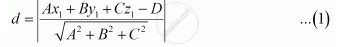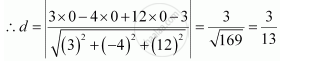Share

# In the Given Cases, Find the Distance of Each of the Given Points from the Corresponding Given Plane. 3x – 4y + 12 Z = 3 - CBSE (Commerce) Class 12 - Mathematics

ConceptDistance of a Point from a Plane

#### Question

In the given cases, find the distance of each of the given points from the corresponding given plane.

Point                    Plane
(0, 0, 0)           3x – 4y + 12 z = 3

#### Solution

It is known that the distance between a point, p(x1y1z1), and a plane, Ax + By + Cz = D, is given by,The given point is (0, 0, 0) and the plane is 3x – 4y + 12 z = 3Is there an error in this question or solution?

#### APPEARS IN

NCERT Solution for Mathematics Textbook for Class 12 (2018 to Current)
Chapter 11: Three Dimensional Geometry
Q: 14.1 | Page no. 494

#### Video TutorialsVIEW ALL 

Solution In the Given Cases, Find the Distance of Each of the Given Points from the Corresponding Given Plane. 3x – 4y + 12 Z = 3 Concept: Distance of a Point from a Plane.
S